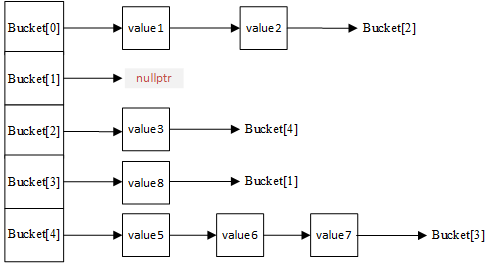vx搜一搜： look_code_art，欢迎关注，会让你相见恨晚

std::unordered_map里面has-a哈希表，它提供的的各个方法基本都是由hashtable封装实现，因此在下文使用hashtable来描述std::unordered_map

  // gnu 实现
template<typename _Key,
typename _Tp,
typename _Hash  = hash<_Key>,
typename _Pred  = equal_to<_Key>,
typename _Alloc = allocator<std::pair<const _Key, _Tp>>>
class unordered_map
{
typedef __umap_hashtable<_Key, _Tp, _Hash, _Pred, _Alloc>  _Hashtable;
_Hashtable _M_h; 	// 内部的唯一成员变量： hashtable
//...
}


### O(1)

1. 计算元素的哈希值。对于单个键值对{key, value}，计算key对应的哈希值 hashcode = hash_func(key)
2. 计算元素在数组中的索引值。由于hashcode不一定处于[0, bucket_count]范围内，因此需要将hashcode映射到该范围：index = hashcode % bucket_count

size_t bucket_index(const std::unordered_map<int, int>&  map, int key) {
size_t bucket_count   = map.bucket_count();		// map 的桶的个数
const auto& hash_func = map.hash_function();	// hash函数
size_t hash_code      = hash_func(key);			// 计算hashcode

// 键key将插入的桶位置
return  hash_code % bucket_count;
}


bucket_index函数时间复杂度是O(1)。在C++中，std::unordered_map提供的bucket(key)方法实现了相同的功能，即计算键key在数组中位置，下面可以验证下bucket_index(...) 的正确性。

int main(int argc, char const *argv[]) {
std::unordered_map<int, int> map(5); // 桶的大小为5
int key = 8;
// 如果 bucket_index(map, key) != map.bucket(key)，
// 会触发 abort 中断
assert( bucket_index(map, key) == map.bucket(key));
// 计算结果
std::cout<<"index: "<< bucket_index(map, key) << std::endl;
}
// 输出
\$ g++ main.cc  -o main && ./main
index: 3


### hash冲突

• 桶的索引值index依然是bucket_index函数的计算方式，即通过待插入节点的键来获取
• 待插入节点的值在哨兵指向的链表头部插入，由于是头部插入整个插入过程还是O(1)时间复杂度。### hash退化

#### 负载因子

    load_factor = map.size() / map.buck_count()	// load_factor 计算方式


• 扩容。即使分配一块更大内存，来容纳更多的桶。
• 重新插入。按照上述插入步骤将原来桶中的buck_size个节点重新插入到新的桶中。

Rehash后，桶数增加了而元素个数不变，再次满足load_factor < max_load_factor条件。

#### Rehash

hashtable，由于要一直满足 load_factor <= max_load_factor ，限制着hash冲突程度，即每个桶的链表节点数不会无限制增加，整个hashtable的节点数达到一定程度就会Rehash，确保hashtable的搜索、删除的平均时间复杂度还是O(1)。

std::unordered_map有个rehash函数，可以在任意时候使std::unordered_map发生Rehash，也可以等load_factor >= max_load_factor时自动发生。注意：

• 不同编译器的扩容策略不同，因此不编译器Rehash后桶的个数不一致很正常。
• 目前msvc和g++中的max_load_factor字段默认值都是1。

///@brief 向 @c map 中添加 n 个int类型值
void insert_n(std::unordered_map<int, int>& map, int start, int n) {

for (int idx = start; idx < start + n; ++idx) {
map.insert({ idx, idx });
}

std::cout << "bucket_cout: " << map.bucket_count()              // 桶的个数
<< "|size: " << map.size()                            // 元素个数
}

int main(int argc, char* const argv[]) {
std::unordered_map<int, int> map;
insert_n(map, 0, 0);    // 初始化状态
insert_n(map, 0, 1);
insert_n(map, 1, 3);
insert_n(map, 3, 4);
insert_n(map, 7, 1);    // 满负载
insert_n(map, 8, 1);    // Rehash

return 0;
}

// 输出如下：